# PID Control Using NI LabVIEW

Publish Date: Feb 11, 2020 | 28 Ratings | 3.54 out of 5 | Print | Submit your review

## Overview

This tutorial shows the characteristics of the proportional (P), the integral (I), and the derivative (D) controls, and how to use them to obtain a desired response. This tutorial uses LabVIEW and the LabVIEW Control Design and Simulation Module.

These tutorials are based on the Control Tutorials developed by Professor Dawn Tilbury of the Mechanical Engineering department at the University of Michigan and Professor Bill Messner of the Department of Mechanical Engineering at Carnegie Mellon University and were developed with their permission.

### 1. The Three-Term Controller

Consider the following unity feedback system: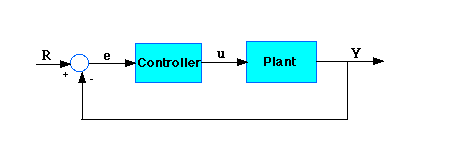Figure 1: Unity Feedback System

Plant: A system to be controlled
Controller: Provides the excitation for the plant; Designed to control the overall system behavior

The transfer function of the PID controller looks like the following: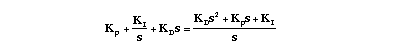• Kp = Proportional gain
• Ki = Integral gain
• Kd = Derivative gain

First, let's take a look at how the PID controller works in a closed-loop system using the schematic shown above. The variable (e) represents the tracking error, the difference between the desired input value (R) and the actual output (Y). This error signal (e) will be sent to the PID controller, and the controller computes both the derivative and the integral of this error signal. The signal (u) just past the controller is now equal to the proportional gain (Kp) times the magnitude of the error plus the integral gain (Ki) times the integral of the error plus the derivative gain (Kd) times the derivative of the error.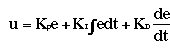This signal (u) will be sent to the plant, and the new output (Y) will be obtained. This new output (Y) will be sent back to the sensor again to find the new error signal (e). The controller takes this new error signal and computes its derivative and its integral again. This process goes on and on.

### 2. The Characteristics of P, I, and D Controllers

A proportional controller (Kp) will have the effect of reducing the rise time and will reduce but never eliminate the steady-state error. An integral control (Ki) will have the effect of eliminating the steady-state error, but it may make the transient response worse. A derivative control (Kd) will have the effect of increasing the stability of the system, reducing the overshoot, and improving the transient response. Effects of each of controllers Kp, Kd, and Ki on a closed-loop system are summarized in the table shown below.

 CL RESPONSE RISE TIME OVERSHOOT SETTLING TIME S-S ERROR Kp Decrease Increase Small Change Decrease Ki Decrease Increase Increase Eliminate Kd Small Change Decrease Decrease Small Change

Figure 2: Effect of PID Controllers on Closed-Loop System

Note that these correlations may not be exactly accurate, because Kp, Ki, and Kd are dependent on each other. In fact, changing one of these variables can change the effect of the other two. For this reason, the table should only be used as a reference when you are determining the values for Ki, Kp and Kd.

### 3. Example Problem

Suppose we have a simple mass, spring, and damper problem.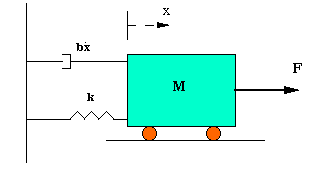Figure 3: Mass, Spring, and Damper

The modeling equation of this system is: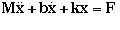Taking the Laplace transform of the modeling equation, we get: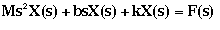The transfer function between the displacement X(s) and the input F(s) then becomes: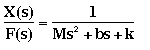Let M = 1kg, b = 10 N.s/m, k = 20 N/m, and F(s) = 1. If we use these values in the above transfer function, the result is: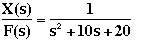The goal of this problem is to show you how each of Kp, Ki and Kd contributes to obtain fast rise time, minimum overshoot, and no steady-state error.

### 4. Open-Loop Step Response

Let's first view the open-loop step response.

### LabVIEW Graphical Approach

Create a new blank VI, and insert the CD Construct Transfer Function Model VI and the CD Draw Transfer Function Equation VI, from the Model Construction section of the Control Design palette.

Create controls for the Numerator and Denominator terminals of the CD Construct Transfer Function Model VI. Connect the Transfer Function Model output from this VI to the input terminal of the CD Draw Transfer Function Equation VI. Finally, create an indicator from the Equation terminal of the CD Draw Transfer Function VI.

Create a While Loop around this code, and create a control for the Loop Condition terminal.

Next, add the CD Step Response VI to the block diagram. Connect the Transfer Function Model output from the CD Construct Transfer Function Model VI to the Transfer Function Model input of the CD Step Response VI. Create an indicator from the Step Response Graph output of the CD Step Response VI.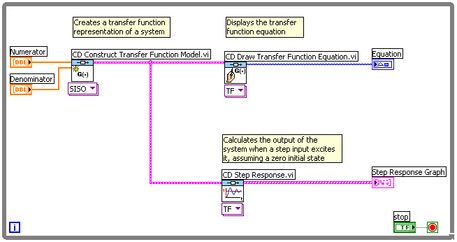### Hybrid Graphical/MathScript Approach

Alternatively, we can use a MathScript Node with the CD Step Response VI to plot the open-loop step response, by using the following code:

plant=tf(num,den);

Make sure to change the MathScript Node output variable data type to TF object.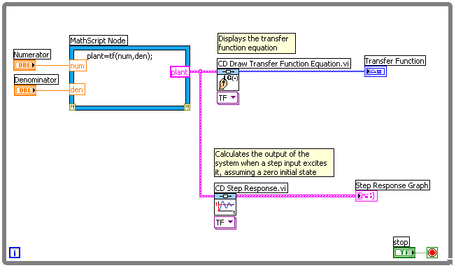### Result

Running the VI from either Figure 4 or Figure 5 should return the plot shown below in Figure 6.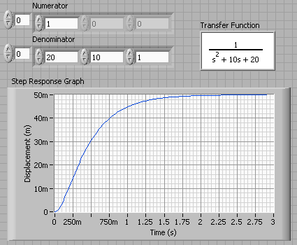Figure 6: Step Response Graph

The DC gain of the plant transfer function is 1/20, so 0.05 is the final value of the output to a unit step input. This corresponds to the steady-state error of 0.95, quite large indeed. Furthermore, the rise time is about one second, and the settling time is about 1.5 seconds. Let's design a controller that will reduce the rise time, reduce the settling time, and eliminates the steady-state error.

### 5. Proportional Control

From the table in Figure 3, we see that the proportional controller (Kp) reduces the rise time, increases the overshoot, and reduces the steady-state error. The closed-loop transfer function of the above system with a proportional controller is: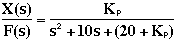### LabVIEW Graphical Approach

Change the CD Construct Transfer Function Model VI to “SISO (Symbolic)” to allow for variables to be used. The resulting block diagram is shown in Figure 7.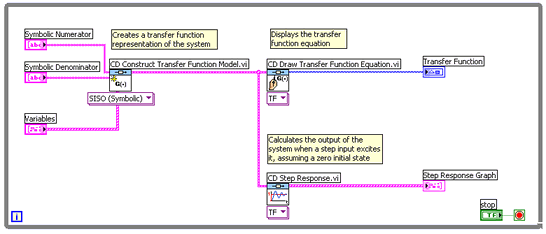Now enter in the closed-loop transfer function of the system with a proportional controller. Let the proportional gain (Kp) equal 300.

### Hybrid Graphical/MathScript Approach

Alternatively, to achieve this result using a MathScript Node, use the following code:

num=1;

den=[1 10 20];

plant=tf(num,den);

Kp=300;

contr=Kp;

sys_cl=feedback(contr*plant,1);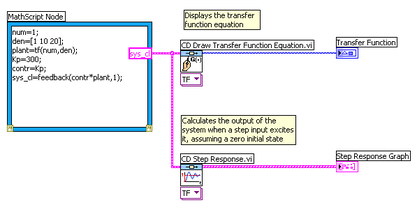Note: The m-file function called feedback was used to obtain a closed-loop transfer function directly from the open-loop transfer function (instead of computing closed-loop transfer function by hand).

### Result

Both the LabVIEW approach and the hybrid approach should yield the graph shown below in Figure 9.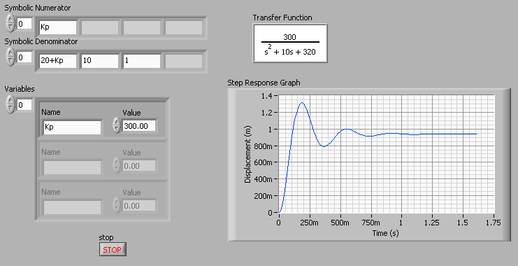Figure 9: Proportional Control

The graph shows that the proportional controller reduced both the rise time and the steady-state error, increased the overshoot, and decreased the settling time by small amount.

### 6. Proportional-Derivative Control

Now, let's take a look at a PD control. From the table in Figure 3, we see that the derivative controller (Kd) reduces both the overshoot and the settling time. The closed-loop transfer function of the given system with a PD controller is: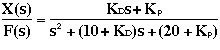Let Kp equal 300 as before and let Kd equal 10.

### LabVIEW Graphical Approach

Using the VI from Figure 7, modify the input terms on the front panel to add the derivative element to the system.

### Hybrid Graphical/MathScript Approach

Alternatively, to achieve this result using a MathScript Node, use the VI from Figure 8 with the following code:

num=1;

den=[1 10 20];

plant=tf(num,den);

Kp=300;

Kd=10;

contr=tf([Kd Kp],1);

sys_cl=feedback(contr*plant,1);

### Result

Both the LabVIEW approach and the hybrid approach should yield the graph shown below in Figure 10.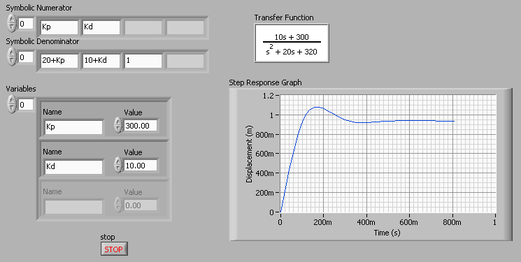Figure 10: Proportional-Derivative Control

Compare the graph in Figure 10 to the graph in Figure 9. The step response plot shows that the derivative controller reduced both the overshoot and the settling time, and had a small effect on the rise time and the steady-state error.

### 7. Proportional-Integral Control

Before going into a PID control, let's take a look at a PI control. From the table, we see that an integral controller (Ki) decreases the rise time, increases both the overshoot and the settling time, and eliminates the steady-state error. For the given system, the closed-loop transfer function with a PI control is: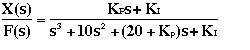Let's reduce the Kp to 30, and let Ki equal 70.

### LabVIEW Graphical Approach

Using the VI from Figure 7, modify the input terms on the front panel to add the derivative element to the system.

### Hybrid Graphical/MathScript Approach

Alternatively, to achieve this result using a MathScript Node, use the VI from Figure 8 with the following code:

num=1;

den=[1 10 20];

plant=tf(num,den);

Kp=30;

Ki=70;

contr=tf([Kp Ki],[1 0]);

sys_cl=feedback(contr*plant,1);

### Result

Both the LabVIEW approach and the hybrid approach should yield the graph shown below in Figure 11.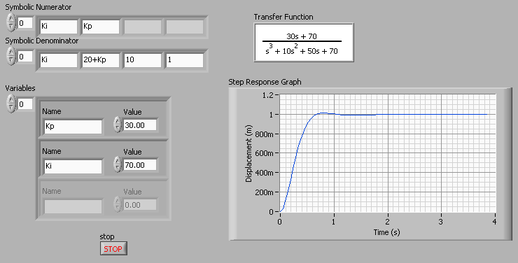Figure 11: Proportional-Integral Control

We have reduced the proportional gain (Kp) because the integral controller also reduces the rise time and increases the overshoot as the proportional controller does (double effect). The above response in Figure 11 shows that the integral controller eliminated the steady-state error.

### 8. Proportional-Integral-Derivative Control

Now, let's take a look at a PID controller. The closed-loop transfer function of the given system with a PID controller is: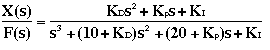After several trial and error runs, the gains Kp=350, Ki=300, and Kd= 50 provided the desired response.< /p> < h3> LabVIEW Graphical Approach< /h3> < p> To confirm, test these terms in your VI, using the VI from Figure 7.< /p> < h3> Hybrid Graphical/MathScript Approach

Alternatively, this result can be achieved with a MathScript Node, by using the VI from Figure 8 with the following code:

num=1;

den=[1 10 20];

plant=tf(num,den);

Kp=350;

Ki=300;

Kd=50;

contr=tf([Kd Kp Ki],[1 0]);

sys_cl=feedback(contr*plant,1);

### Result

Both the LabVIEW approach and the hybrid approach should yield the graph shown below in Figure 12.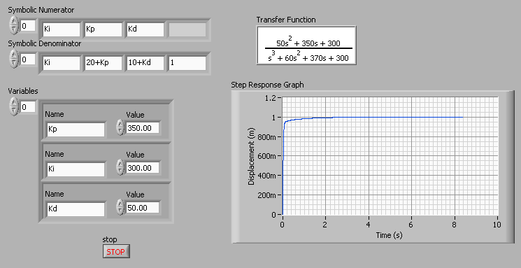Figure 12: Proportional-Integral-Derivative Control

Now, we have obtained a closed-loop system with no overshoot, fast rise time, and no steady-state error.

### 9. General Tips for Designing a PID Controller

When you are designing a PID controller for a given system, follow the steps shown below to obtain a desired response.

1. Obtain an open-loop response and determine what needs to be improved
2. Add a proportional control to improve the rise time
3. Add a derivative control to improve the overshoot
5. Adjust each of Kp, Ki, and Kd until you obtain a desired overall response. You can always refer to the table shown in this "PID Tutorial" page to find out which controller controls what characteristics.

Keep in mind that you do not need to implement all three controllers (proportional, derivative, and integral) into a single system, if not necessary. For example, if a PI controller gives a good enough response (like the above example), then you don't need to implement a derivative controller on the system. Keep the controller as simple as possible.